# RD Sharma Solutions for Class 9 Maths Chapter 19 Surface Area and Volume of A Right Circular Cylinder Exercise 19.1

Class 9 Chapter 19 – Surface Area and Volume of A Right Circular Cylinder Exercise 19.1 solutions are provided here. This exercise deals with surface area of a cylinder. Practice problems on surface areas of a circular cylinder through solved RD Sharma solutions to have a better understanding of the topic. The solutions that are provided here have been prepared by subject experts to further help students boost their scoring potential in the examination. Click on the link below to get your pdf now.

## Download PDF of RD Sharma Solutions for Class 9 Maths Chapter 19 Surface Area and Volume of A Right Circular Cylinder Exercise 19.1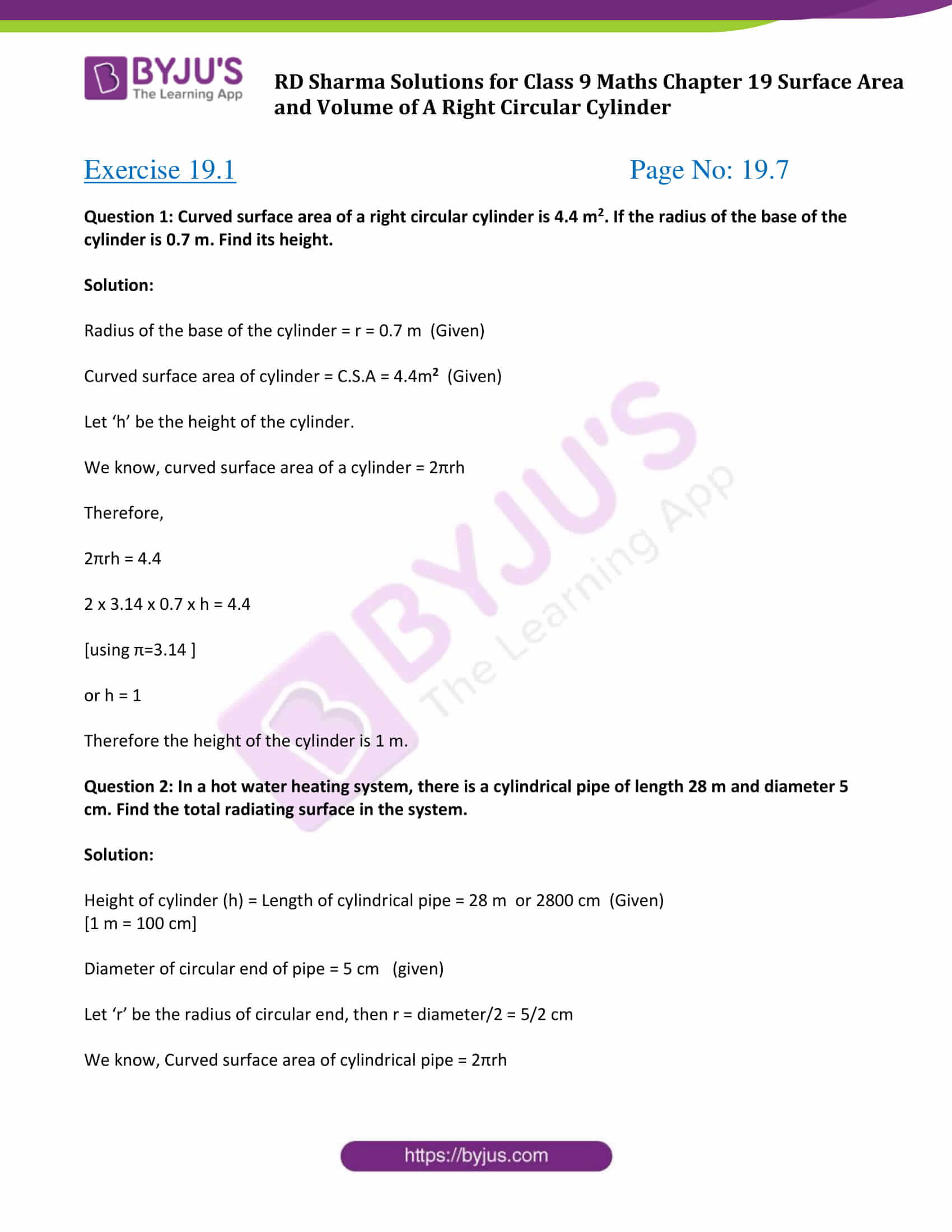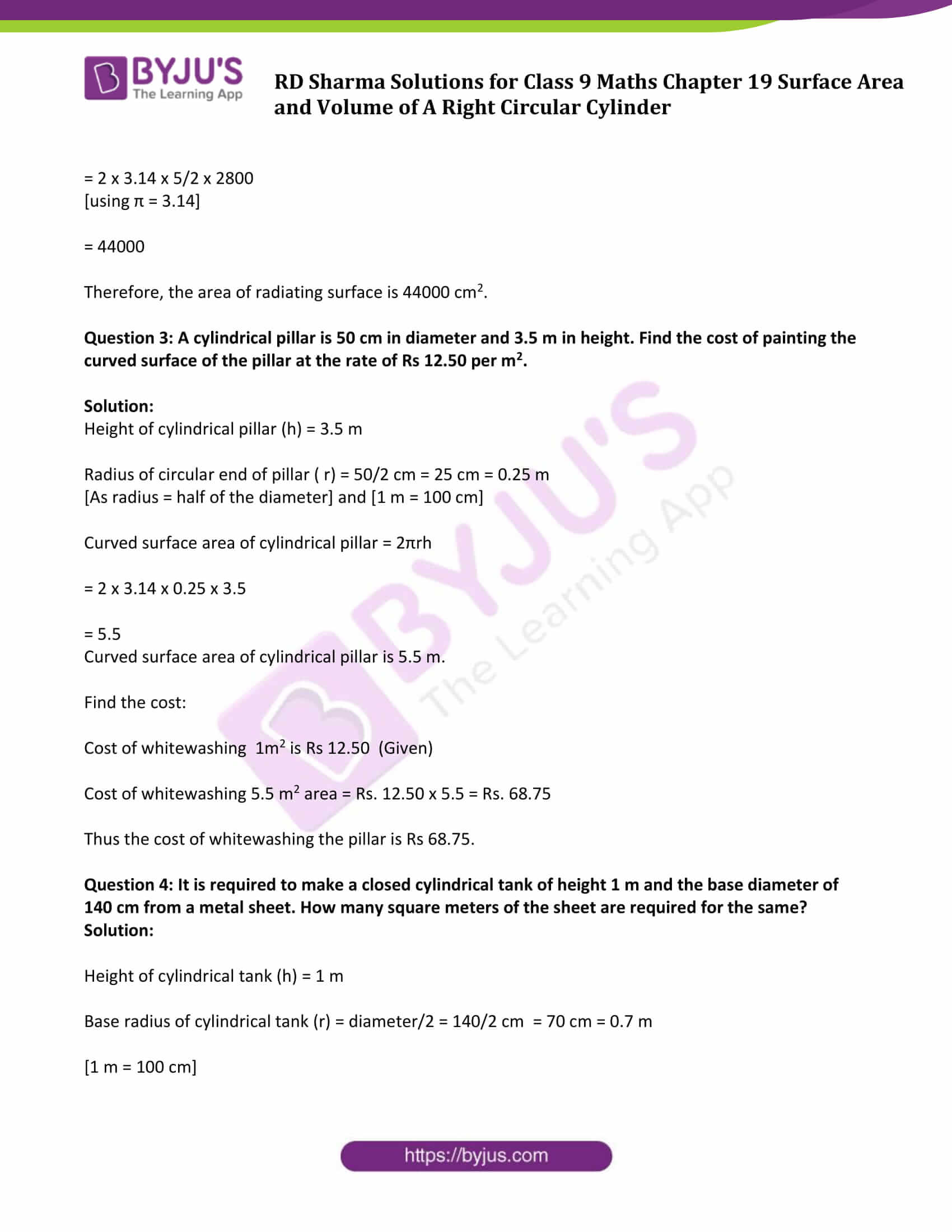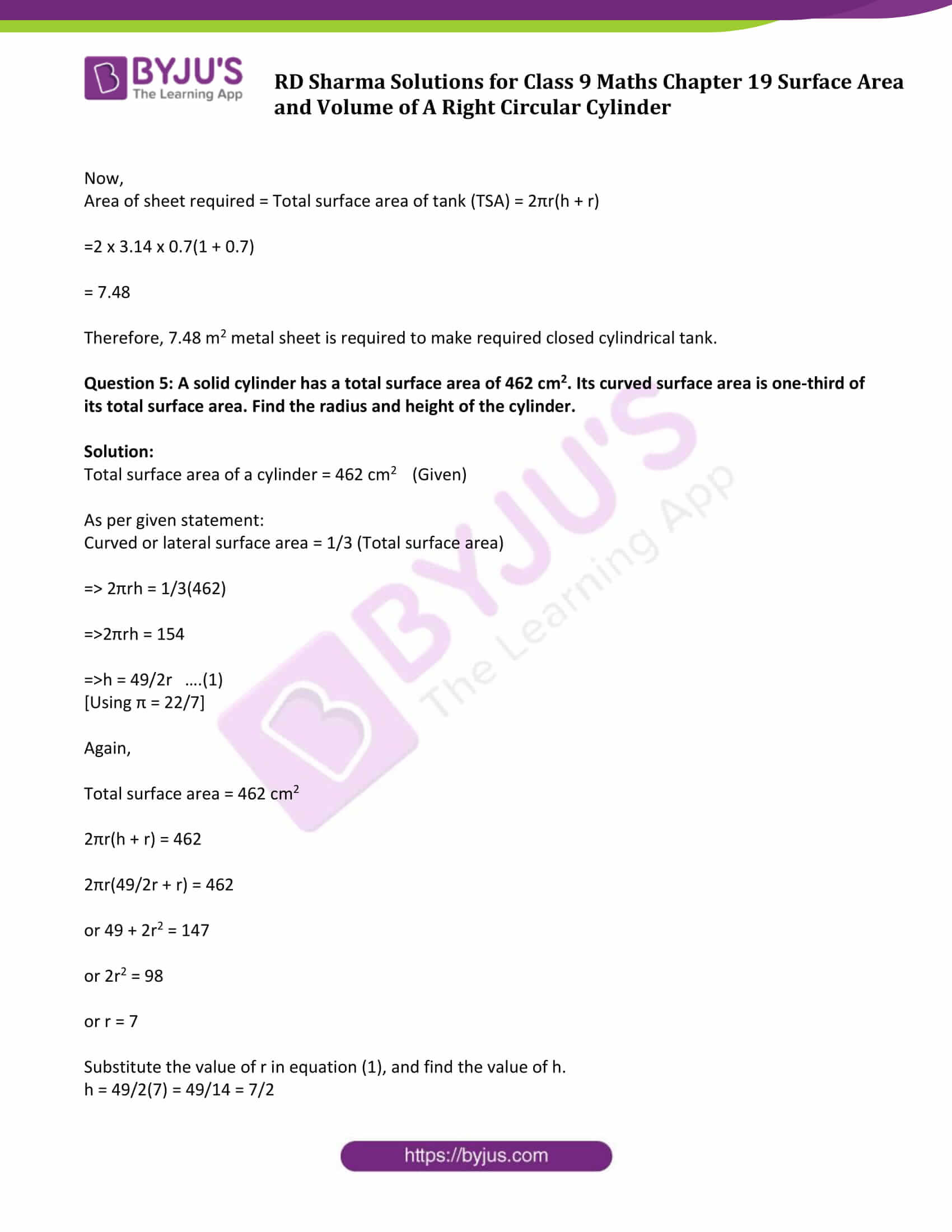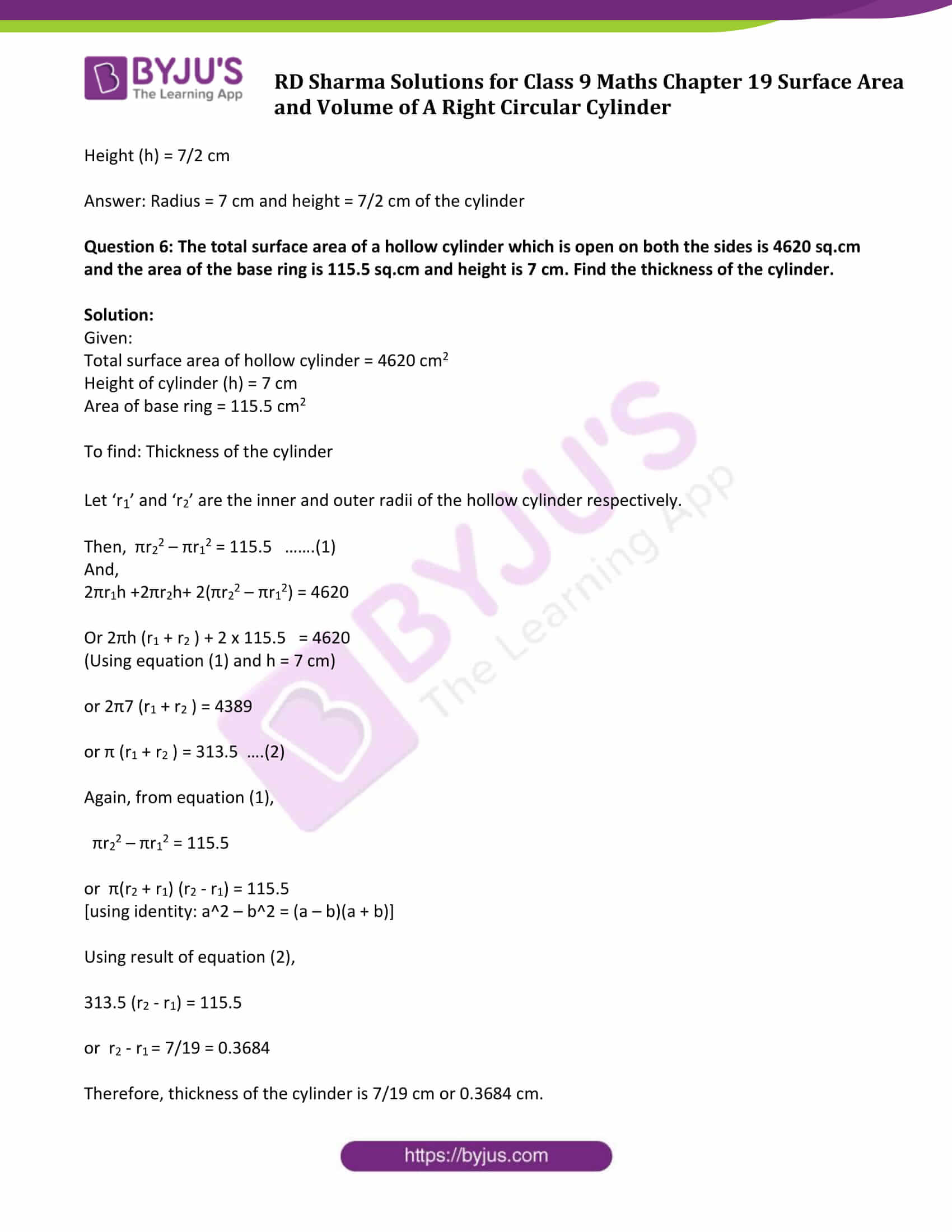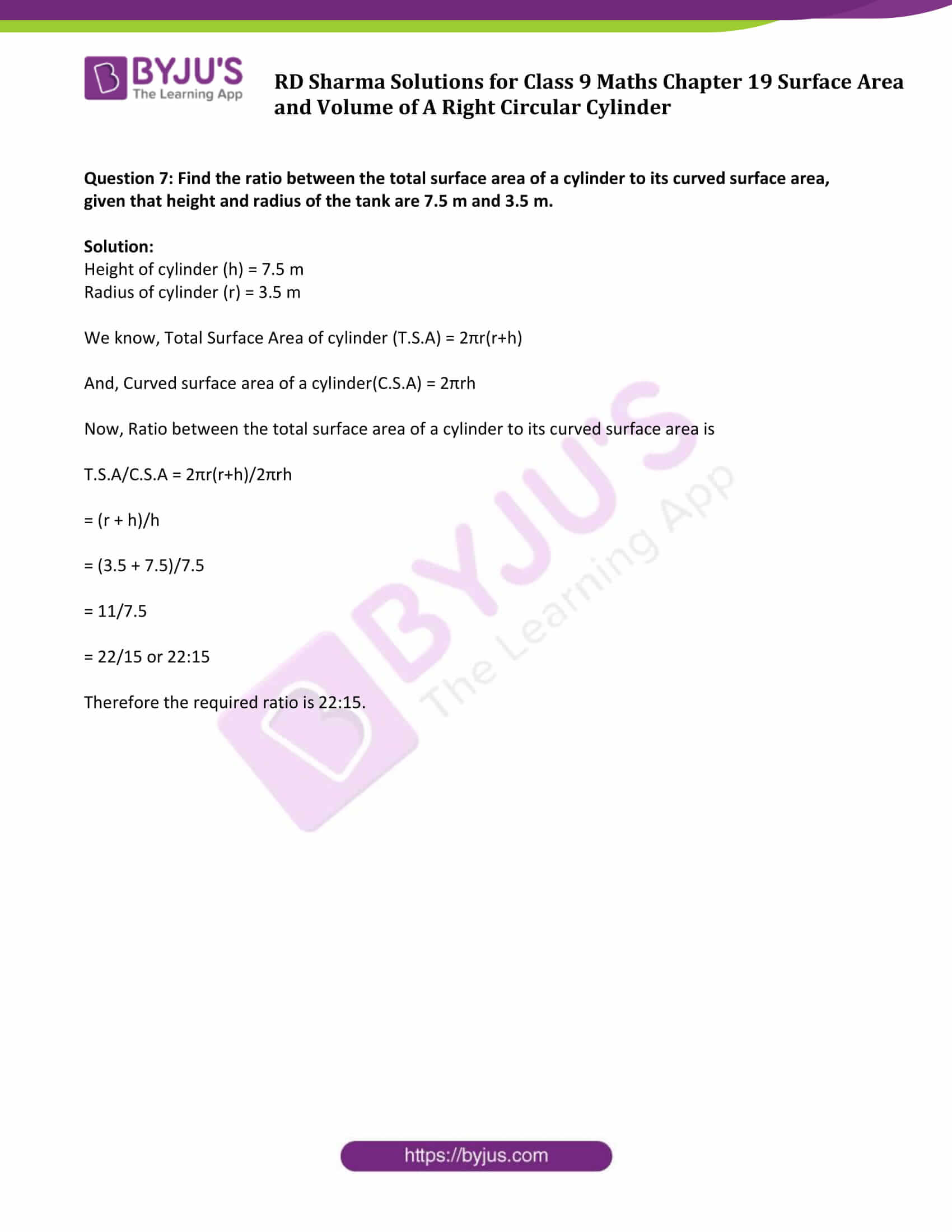## Exercise 19.1 Page No: 19.7

Question 1: Curved surface area of a right circular cylinder is 4.4 m2. If the radius of the base of the cylinder is 0.7 m. Find its height.

Solution:

Radius of the base of the cylinder = r = 0.7 m (Given)

Curved surface area of cylinder = C.S.A = 4.4m2 (Given)

Let ‘h’ be the height of the cylinder.

We know, curved surface area of a cylinder = 2πrh

Therefore,

2πrh = 4.4

2 x 3.14 x 0.7 x h = 4.4

[using π=3.14 ]

or h = 1

Therefore the height of the cylinder is 1 m.

Question 2: In a hot water heating system, there is a cylindrical pipe of length 28 m and diameter 5 cm. Find the total radiating surface in the system.

Solution:

Height of cylinder (h) = Length of cylindrical pipe = 28 m or 2800 cm (Given)

[1 m = 100 cm]

Diameter of circular end of pipe = 5 cm (given)

Let ‘r’ be the radius of circular end, then r = diameter/2 = 5/2 cm

We know, Curved surface area of cylindrical pipe = 2πrh

= 2 x 3.14 x 5/2 x 2800

[using π = 3.14]

= 44000

Therefore, the area of radiating surface is 44000 cm2.

Question 3: A cylindrical pillar is 50 cm in diameter and 3.5 m in height. Find the cost of painting the curved surface of the pillar at the rate of Rs 12.50 per m2.

Solution:

Height of cylindrical pillar (h) = 3.5 m

Radius of circular end of pillar ( r) = 50/2 cm = 25 cm = 0.25 m

[As radius = half of the diameter] and [1 m = 100 cm]

Curved surface area of cylindrical pillar = 2πrh

= 2 x 3.14 x 0.25 x 3.5

= 5.5

Curved surface area of cylindrical pillar is 5.5 m.

Find the cost:

Cost of whitewashing 1m2 is Rs 12.50 (Given)

Cost of whitewashing 5.5 m2 area = Rs. 12.50 x 5.5 = Rs. 68.75

Thus the cost of whitewashing the pillar is Rs 68.75.

Question 4: It is required to make a closed cylindrical tank of height 1 m and the base diameter of 140 cm from a metal sheet. How many square meters of the sheet are required for the same?

Solution:

Height of cylindrical tank (h) = 1 m

Base radius of cylindrical tank (r) = diameter/2 = 140/2 cm = 70 cm = 0.7 m

[1 m = 100 cm]

Now,

Area of sheet required = Total surface area of tank (TSA) = 2πr(h + r)

=2 x 3.14 x 0.7(1 + 0.7)

= 7.48

Therefore, 7.48 m2 metal sheet is required to make required closed cylindrical tank.

Question 5: A solid cylinder has a total surface area of 462 cm2. Its curved surface area is one-third of its total surface area. Find the radius and height of the cylinder.

Solution:

Total surface area of a cylinder = 462 cm2 (Given)

As per given statement:

Curved or lateral surface area = 1/3 (Total surface area)

⇒ 2πrh = 1/3(462)

⇒ 2πrh = 154

⇒ h = 49/2r ….(1)

[Using π = 22/7]

Again,

Total surface area = 462 cm2

2πr(h + r) = 462

2πr(49/2r + r) = 462

or 49 + 2r2 = 147

or 2r2 = 98

or r = 7

Substitute the value of r in equation (1), and find the value of h.

h = 49/2(7) = 49/14 = 7/2

Height (h) = 7/2 cm

Answer: Radius = 7 cm and height = 7/2 cm of the cylinder

Question 6: The total surface area of a hollow cylinder which is open on both the sides is 4620 sq.cm and the area of the base ring is 115.5 sq.cm and height is 7 cm. Find the thickness of the cylinder.

Solution:

Given:

Total surface area of hollow cylinder = 4620 cm2

Height of cylinder (h) = 7 cm

Area of base ring = 115.5 cm2

To find: Thickness of the cylinder

Let ‘r1’ and ‘r2’ are the inner and outer radii of the hollow cylinder respectively.

Then, πr22 – πr12 = 115.5 …….(1)

And,

2πr1h +2πr2h+ 2(πr22 – πr12) = 4620

Or 2πh (r1 + r2 ) + 2 x 115.5 = 4620

(Using equation (1) and h = 7 cm)

or 2π7 (r1 + r2 ) = 4389

or π (r1 + r2 ) = 313.5 ….(2)

Again, from equation (1),

πr22 – πr12 = 115.5

or π(r2 + r1) (r2 – r1) = 115.5

[using identity: a^2 – b^2 = (a – b)(a + b)]

Using result of equation (2),

313.5 (r2 – r1) = 115.5

or r2 – r1 = 7/19 = 0.3684

Therefore, thickness of the cylinder is 7/19 cm or 0.3684 cm.

Question 7: Find the ratio between the total surface area of a cylinder to its curved surface area, given that height and radius of the tank are 7.5 m and 3.5 m.

Solution:

Height of cylinder (h) = 7.5 m

Radius of cylinder (r) = 3.5 m

We know, Total Surface Area of cylinder (T.S.A) = 2πr(r+h)

And, Curved surface area of a cylinder(C.S.A) = 2πrh

Now, Ratio between the total surface area of a cylinder to its curved surface area is

T.S.A/C.S.A = 2πr(r+h)/2πrh

= (r + h)/h

= (3.5 + 7.5)/7.5

= 11/7.5

= 22/15 or 22:15

Therefore the required ratio is 22:15.

## RD Sharma Solutions for Class 9 Maths Chapter 19 Surface Area and Volume of A Right Circular Cylinder Exercise 19.1

RD Sharma Solutions Class 9 Maths Chapter 19 Surface Area and Volume of A Right Circular Cylinder Exercise 19.1 is based on the following topics:

• Surface Area of a Right Circular Cylinder
• Surface Area of a Hollow Cylinder